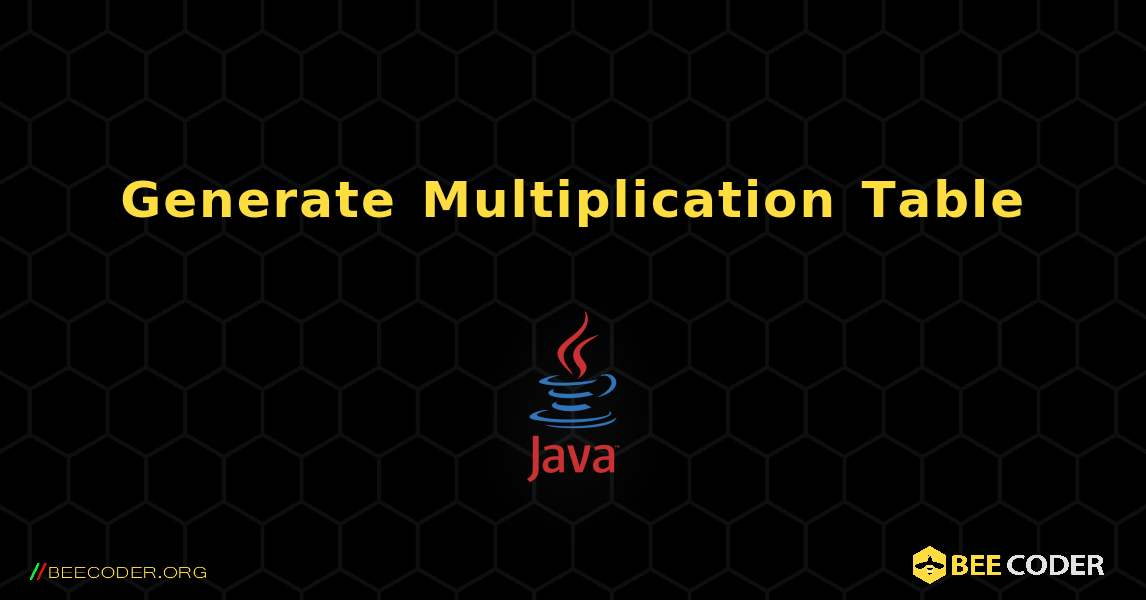# Generate Multiplication Table. Java

`Generate Multiplication Table using for loop`Java

Output result
```5 * 1 = 5
5 * 2 = 10
5 * 3 = 15
5 * 4 = 20
5 * 5 = 25
5 * 6 = 30
5 * 7 = 35
5 * 8 = 40
5 * 9 = 45
5 * 10 = 50```
`Generate Multiplication Table using while loop`Java

Output result
```9 * 1 = 9
9 * 2 = 18
9 * 3 = 27
9 * 4 = 36
9 * 5 = 45
9 * 6 = 54
9 * 7 = 63
9 * 8 = 72
9 * 9 = 81
9 * 10 = 90```## BeeCoder.ORG

###### open source code and examples

We have been online since 2021 and 1 millions of people around the globe have visited our website since then

More visitors every month